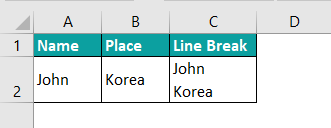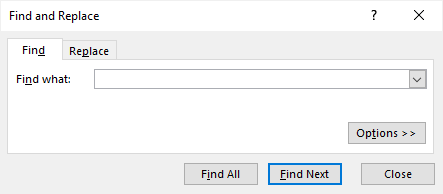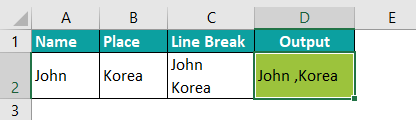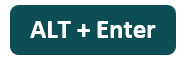# Line Breaks in ExcelArticle byExcelMojo Team## What Is A Line Break In Excel Cell?

The Line Break in Excel Cell inserts a new line in the cell value. It breaks the text string into multiple parts and helps users show it in separate lines. The keyboard shortcut of the Line Break in Excel Cell is ALT + ENTER. As we insert a line break, the cell’s height also increases as it represents the data in the cell.

For example, the below table shows an address. We want to show each part of the address in a separate line. Therefore, we need to use the below steps to insert line breaks.

Step 1: Select where we want to break the line.

Step 2: Press ALT + ENTER.

Clearly, we can see that the text strings into multiple lines, as shown in the image below:

Likewise, we can add line breaks in worksheets.

###### Key Takeaways
• The Line Breaks in Excel is a feature that breaks the text string into multiple lines within a cell.
• There are two ways to insert line breaks in Excel. They are using the keyboard shortcut or a formula.
• The shortcut keys to insert line breaks in Excel are Alt + Enter, and the shortcut keys to remove line breaks are Ctrl + J.
• The keyboard shortcut enables the Wrap Text automatically.
• Excel does not enable the Wrap Text when we use the formula to add a line break. We have to enable it manually.

### How To Insert Line Break In Excel Cell?

We have multiple methods to add line breaks. They are:

• By using the keyboard shortcut
• Using & formula
• Using Concatenate Formula
• Using Conventional Approach

Let us see how to insert line breaks in Excel using these methods.

#### #1 – Line Break In Excel Cell (Alt+Enter)

The table below contains Ron’s details, such as his full name and address. Next, we need to use the following steps to insert Line Breaks using the keyboard shortcuts ALT + Enter.

In the table,

• Columns A and B show the first and last names.
• Columns C, D, E, and F display the street, city, state, and postal code respectively.

The steps used to insert line breaks using the keyboard shortcuts ALT + Enter are as follows:

Step 1: Select an empty cell to display the output. We have selected cell G2 in this case.

Step 2: Enter the full address with the full name.

Step 3: Place the cursor where we want to break the line and press the shortcut keys ALT + Enter.

Clearly, we can see that the text is broken into multiple lines.

Similarly, we can insert line breaks.

#### #2 – Line Break With Excel Formula (& Function)

The following table shows the occupations of various people along with the location. We need to use the below steps to insert line breaks in worksheet.

In the table,

• Column A shows the name.
• Column B displays the occupation.
• Column C indicates the place.

The steps used to add line breaks using the & Function are as follows:

Step 1:  Select an empty cell to display the output. We have selected cell D2 in this case.

Step 2:  Next, start by entering the formula in cell D2.

Step 3: We use the & function with the CHAR function.

So, the complete formula is =A2&CHAR(10)&B2&CHAR(10)&C2&CHAR(10).

Step 4: Press Enter key.

Excel returns the output of the formula as shown in the image below:

Step 5: Now, we can manually enter the formulas or use the AutoFill option.

In this example, let us use the AutoFill option.

Drag the formula downwards till cell D5.

We have obtained the required result.

Similarly, we can easily break the text string using line breaks.

#### #3.1 – Line Break With Excel Formula (Concatenate)

The below table shows students’ names and subjects in columns A and B, respectively. We need to insert line breaks in excel using the CONCATENATE Excel function combining cells with commas.

The steps used to insert line breaks using the CONCATENATE function are as follows:

Step 1: Select an empty cell to display the output. We have selected cell C2 in this case.

Step 2:  Next, start by entering the formula in cell C2.

Step 3: The CONCATENATE function is =CONCATENATE(A2,”,”, B2).

The function combines the string and presents it in the line, but the line break feature breaks the string in different lines in the same cell.

Step 4: Press Enter key.

We can see that the text is broken into two lines in the same cell.

Step 5: Using AutoFill, drag the formula downwards till cell D7.

Likewise, we can insert line breaks using the CONCATENATE function.

#### #3.2 – Line Break with Excel Formula (Concatenate)

The following table shows the phone numbers of various people in columns A and B. We need to use the below steps to insert line breaks using the CONCATENATE function combining cells with commas.

The steps used to insert line breaks using the CONCATENATE function are as follows:

Step 1:  Select an empty cell to display the output. We have selected cell C2 in this case.

Step 2:  Next, start by entering the formula in cell C2.

Step 3: The complete CONCATENATE formula is

=CONCATENATE(A2,“ ”,“Phone No. is”,“ ”, B2).

The function combines the string and presents it in the line, but the line breaks option breaks the string in different lines in the same cell.

Step 4: Press Enter key.

The Line Break Cell technique is shown in cell D2.

Step 5: Press Enter key. Drag the formula downwards till cell D5 to obtain the results.

Similarly, we can insert line breaks with CONCATENATE function.

#### #4 – Line Break with Excel Cell (Conventional way)

Consider the following text string in cell A1. We need to insert line breaks using the keyboard shortcuts ALT + Enter conventionally.

The steps used to insert line breaks using the keyboard shortcuts ALT + Enter are as follows:

Step 1: Select an empty cell to display the output. We have selected cell A2 in this case.

Step 2: Enter the string in cell A2.

Step 3: Press ALT + Enter keys after each word, as shown in the following image.

We can see that the text is broken into multiple lines.

Likewise, we can use line breaks to break text strings.

### Insert A Line Break After A Specific Character

The following table shows the name of the fruit in cell A2. Now, we need to insert line breaks after a specific character.

The steps used to insert line breaks after a specific character are as follows:

Step 1: Select an empty cell to display the output. We have selected cell B2 in this case.

Step 2: Enter the fruits in cell B2.

Step 3: Press ALT + Enter shortcut keys after the character ‘e.’

For example, use the shortcut keys after the word ‘Pine’ as shown in the following image.

Likewise, we can also insert line breaks after a specific character.

### Important Things To Note

• We can break a text string into lines using the shortcut keys or the formula.
• The Line Breaks in Excel uses ‘&,’ ‘CHAR,’ and ‘CONCATENATE’ functions.
• CHAR function returns the result based on a character code.
• The shortcut keys to use break lines feature is ‘Alt + Enter’ but this method works only for a cell in Excel. We must repeat the process to obtain results in other cells.
• The line breaks in Excel feature enable the Wrap Text option automatically.

How to remove Line Break in Excel Cell?

We can remove line breaks in excel using the below steps.

Consider the following table with the name and place of a person in columns A and B, respectively. Cell C2 shows the output of line breaks in the excel feature. Now, we need to use the following steps to understand how to remove line breaks in excel.The steps to remove line breaks in excel are as follows:

Step 1: Select an empty cell to display the output. We have selected cell D2 in this case.

Step 2: Go to the Home tab, then select the Find & Select option from the Editing group. Click the drop-down arrow, and select the Replace… option.

Step 3: The Find and Replace window pops up.Place the cursor on the Find what: box, press CTLR + J, and enter ‘,’ in the Replace with: box.

Step 4: Click on the Replace button.

Step 5: The Line Break in Excel Cell gets removed, as shown in cell D2.Thus, we now know how to remove line breaks in excel.

How to find Line Break in Excel Cell?

To find line breaks in excel,

1. Select the cell with the text string.
2. Place the cursor on the line break and double-click.

If the line break in excel is not present, the cell will take the width of the string, but if the line break in excel is present, the series will be in the line break feature.

What is the keyboard shortcut key of the Line Break in Excel Cell?

The shortcut keys of line break in excel are: